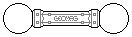Web textodigital.com
Concentric icosidodecahedra
In this construction, we build a rhombitruncated icosidodecahedron around a rhombicosidodecahedron. We'll use the first reinforced version, as I guess that this construction, performed as shown, wouldn't be possible using a normal one.
An icosidodecahedron can be built on any of the pentagonal faces of a rhombicosidodecahedron. The «equator», made in this case of yellow rods, is a regular decagon which exactly matches distance and orientation with a decagonal face of a rhombitruncated icosidodecahedron concentric with the core rhombicosidodecahedron: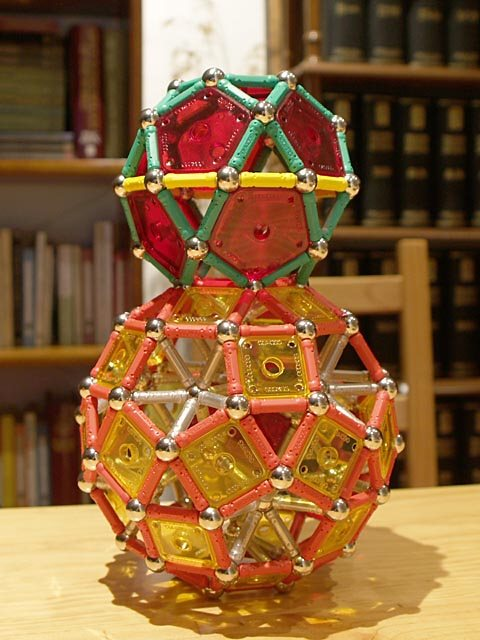Construction of the rhombitruncated icosidodecahedron around the rhombicosidodecahedron, step 1
So, we leave one half icosidodecahedron and proceed to build another five halves on the five pentagonal faces around it: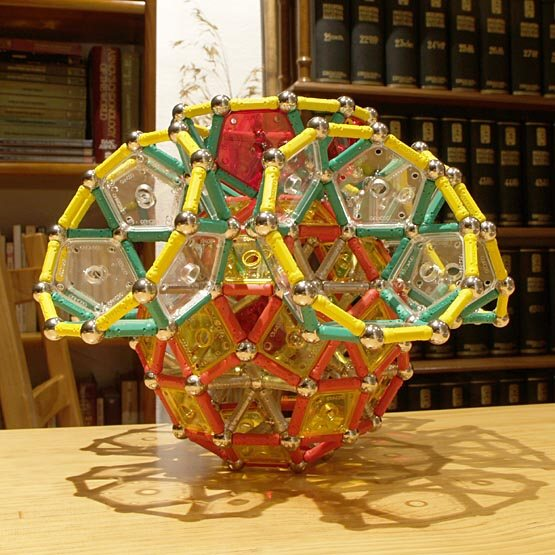Construction of the rhombitruncated icosidodecahedron around the rhombicosidodecahedron, step 2
We form the square and hexagonal faces of the rhombitruncated icosidodecahedron by joining each pair of adjacent decagons with two rods: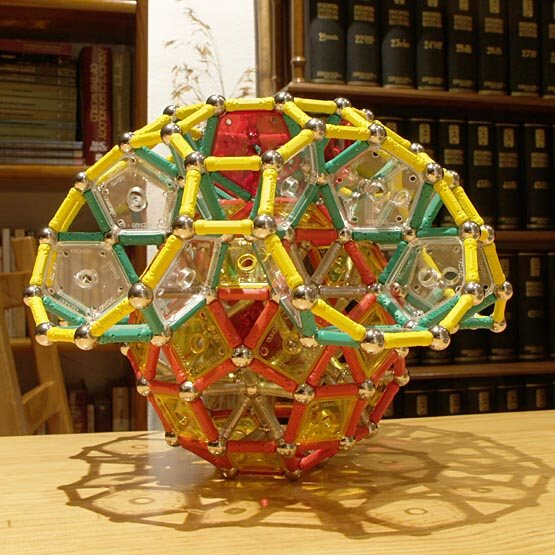Construction of the rhombitruncated icosidodecahedron around the rhombicosidodecahedron, step 3
However, these square and hexagonal faces don't require stabilization by panels or additional rods, as the central rhombicosidodecahedron and the halved icosidodecahedra provide enough strength and rigidity. In fact, we can flip the whole thing upside down quite easily: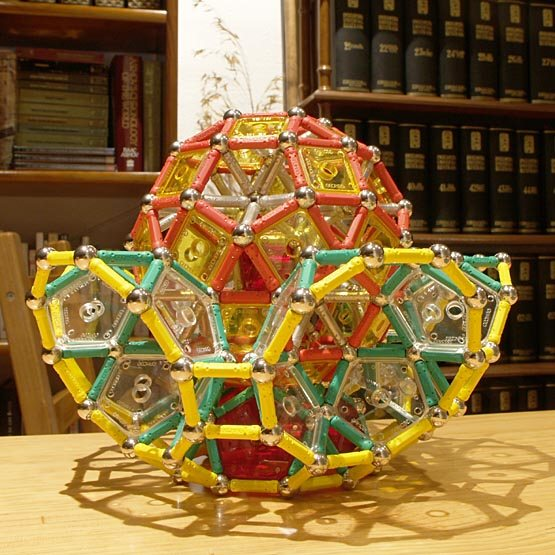Construction of the rhombitruncated icosidodecahedron around the rhombicosidodecahedron, step 4
Just by building another six half-icosidodecahedra, and not forgetting to join each pair of decagons, it is done: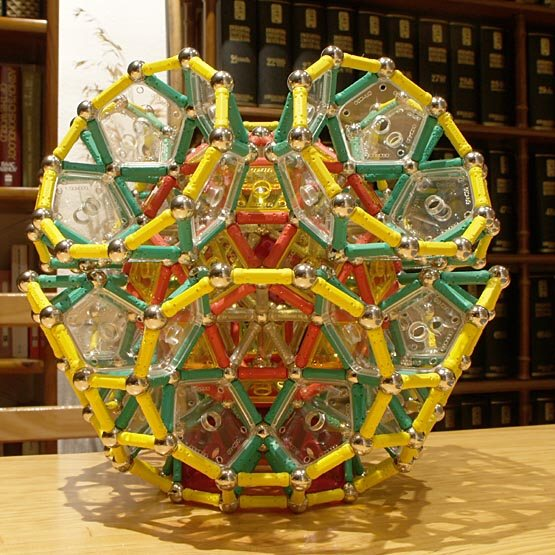Construction of the rhombitruncated icosidodecahedron around the rhombicosidodecahedron, step 5Rhombitruncated icosidodecahedron around the rhombicosidodecahedron 992 pieces: 260 balls, 636 rods, 60 pentagons, 36 squares (5.47 kg)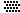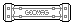The height of a half icosidodecahedron is the same as that of an icosahedron with a pentagonal pyramid removed:
Building a regular decagon around the latter is easy, and it is at the same height as its top vertex:
So, we can use pieces like the right-hand one in the previous picture to build the decagons of the rhombitruncated icosidodecahedron. This time we used the second version of the reinforced rhombicosidodecahedron: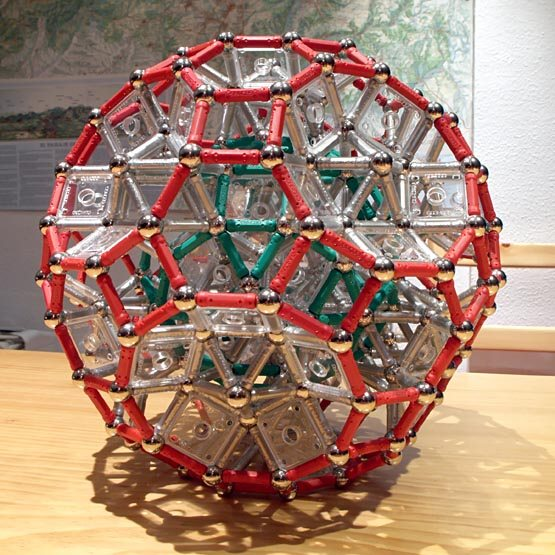Rhombitruncated icosidodecahedron around the rhombicosidodecahedron, version 2 1112 pieces: 272 balls, 756 rods, 24 pentagons, 60 squares (6.07 kg)